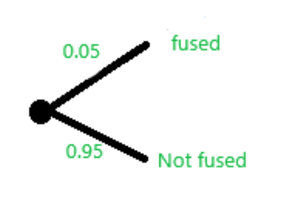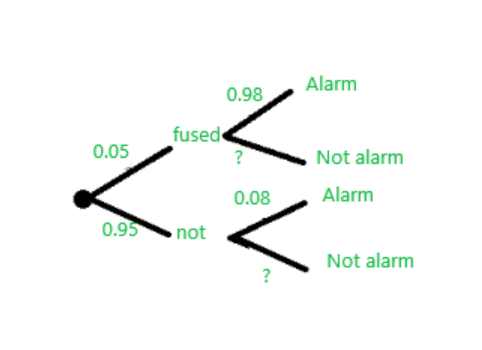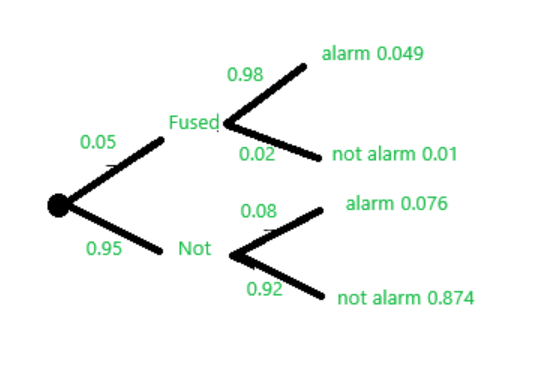Conditional Probability and Independence – Probability | Class 12 Maths

• Last Updated : 16 Mar, 2021

Let B be an event with non-zero probability. The conditional probability of any event A given B is defined as:In other words, P(A|B) is the probability measure of event A after observing the occurrence of event B. Two events are called independent if and only if P(A∩B) = P(A)P(B) (or equivalently, P(A|B) = P(A)). Therefore, independence is equivalent to saying that observing B does not have any effect on the probability of A.

Examples

Example 1: Sapan took part in two games. The probability of her passing both games is 0.4 The probability of her passing the first game is 0.6. What is the probability of her passing the second game given that she has passed the first game?

Solution:

Let’s say 1st game be first and 2nd game be second.

P(first ∩ second) = 0.4
P(first) = 0.6

Formula:Here question is which is right way to write it P(first/second) or P(second/first).

we have given the probability of passing the first test as the definition of conditional probability say The probability of an event occurring given that another event has already occurred is called a conditional probability. Hence, Putting the probabilities into the formula.= 0.66

Example 2: In a group of 100 sports car buyers, 40 bought alarm systems, 30 purchased bucket seats, and 20 purchased an alarm system and bucket seats. If a car buyer chosen at random bought an alarm system, what is the probability they also bought bucket seats?

Solution:

Let’s say event of buying alarm system as alarm and event of buying bucket seats as bucket.

P(alarm) = 40 / 100 = 0.4
P(alarm ∩ bucket) = 0.2

Applying formula: P(A|B) =  P(A∩B) / P(B)

Here we have the probability of alarm systems. and have to find out the probability of bucket seats. Hence, Putting the probabilities into the formula.= 0.5

Example 3: There are 2 red shirts, 4 blue shirts, and 9 white shirts in a basket. Two shirts are randomly selected. Find the probability that the second shirt is red given that the first shirt is blue. (Assume that the first chip is not replaced).

Solution:

Let A be the event blue shirt is selected and not replaced and B be the event second shirt is red.

P(A) = 4 / 15 = 0.26

So blue shirt is already taken and not replaced We left with 14 shirts.

P(A∩B) = 2 / 14 = 0.14

Applying formula:

P(B|A) = P(A∩B) / P(A) = 0.14 / 0.26 = 0.53

Example 4: A coin is flipped twice. Let A be the event that both flip land on heads and let B be the event that at least one flip lands on heads.

Solution:

Sample space of an experiment or random trial is the set of all possible outcomes or results of that experiment. Here ‘S’ denotes the sample space.

S = {(H, H), (H, T), (T, H), (T, T)}

P(A∩B) = 1 / 4 = 0.25

P(B) = 3 / 4 = 0.75

Applying formula:= 0.33

Conditional Probability using Two-way Tables

A survey is conducted in a college on which people were asked what they would like to watch. This two-way table displays data for the sample of students who responded to the survey:

Question: Find the probability that the student was a girl, given that the student voted for Harry Potter.

Solution:

This question can be divided into two parts:

1. Find the probability that the student chooses Harry Potter as the series they would like to watch?= 0.7

2. Find the probability of students that the student was a girl and voted for harry potter= 0.25

Applying Conditional Probability formula:

P(girl | harry potter) == 0.25

Tree Diagram and Conditional Probability

A company needs to check whether a bulb is fused or not and an alarm is triggered when a fused bulb is detected. Suppose that 5% of sets contain a fused bulb. If a set of bulbs contains a fused bulb there is a 98% chance that it triggers the alarm. if a set doesn’t contain a fused bulb, there is an 8% chance that it triggers the alarm. A randomly chosen set of bulbs is taken, what is the probability that it contains a forbidden item? Probability of randomly choosing a set that contains a fused bulb:What is the probability that a randomly chosen bag does NOT contain a forbidden item?

P(not fused) = 1 − P(fused) = 1 − 0.05 = 0.95

If a set contains a fused bulb,there is 98% of chance being detected. If a bag doesn’t contain a fused item, there is 8% chance that it triggers the alarm.Given that a set contains a fused item, what is the probability that it does NOT trigger the alarm?

P(contains the fused bulb not trigger the alarm) = 1 − 0.98 = 0.02

Given that a set does NOT contain a fused item, what is the probability that it does NOT trigger the alarm?

P(does not contain fused and don’t trigger) = 1 − 0.08 = 0.92

Complete tree diagram:Conditional Question: Given a randomly chosen set triggers the alarm, what is the probability that it contains a fused bulb?

P(fused ∣ triggers the alarm) = P(F∩A) / P(A)

We found this probability in the tree diagram. Since 5%, percent of bags contain a fused item, and 98%, of those bags, trigger the alarm, we can multiply those probabilities:

P(F∩A) = (0.05) ∗ (0.98) = 0.049

Find the probability that a randomly selected set triggers the alarm. There are two situations where sets can trigger the alarm, so we add those two probabilities together:

P(A) = P(F∩A) + P(N∩A) = 0.049 + 0.076 = 0.125​

Final result:

P(F∣A) = P(F∩A) / P(A) = 0.049 / (0.049 + 0.076) = 0.049 / 0.125 = 0.392

Analyzing Event Probability for Independence

Tom’s has 1 blue shirt, 1 green shirt, 1 blue hat, 1 green scarf. 1 blue pair of pants. and 1 green pair of pants Tom selects one of these garments at random. Let A be the event of selecting a blue garment and B be the event that chooses a shirt.

P(A) = 3 / 6 = 1 / 2

P(B) = 2 / 6 = 1 / 3

P(A/B) = 1 / 2
P(B/A) = 1 / 3
P(A∩B) = 1 / 6

Verification:

• P(B/A) = P(B) * P(A)

1/6 = 1/6

• P(B/A) = P(B)

1/3 = 1/3

P(A/B) = P(A)  It is the probability of choosing a blue garment given that he chooses a shirt is equal to the probability that tom selects a blue garment.

P(B/A) = P(B), the probability that tom selects a shirt given that he has chosen a blue garment is equal to the probability that tom selects a shirt.

Remember the formula of the independent above i.e.

P(A∩B) = P(A) ∗ P(B)

P(A/B) = P(A)

My Personal Notes arrow_drop_up